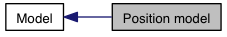Position model
Collaboration diagram for Position model:The position model simulates a mobile robot base. It can drive in one of two modes; either differential, i.e. able to control its speed and turn rate by driving left and roght wheels like a Pioneer robot, or omnidirectional, i.e. able to control each of its three axes independently.

## Worldfile properties

Summary and default values
```    position
(
# position properties
drive "diff"

velocity [ 0.0 0.0 0.0 0.0 ]

localization "gps"
localization_origin [ <defaults to model's start pose> ]

# odometry error model parameters,
# only used if localization is set to "odom"
odom_error [0.03 0.03 0.00 0.05]

# only used if drive is set to "car"
wheelbase 1.0

# [ xmin xmax ymin ymax zmin zmax amin amax ]
velocity_bounds [-1 1 -1 1 -1 1 -90 90 ]
acceleration_bounds [-1 1 -1 1 -1 1 -90 90]

# model properties

)
```
Note
Since Stage-1.6.5 the odom property has been removed. Stage will generate a warning if odom is defined in your worldfile. See localization_origin instead.
Details
• acceleration_bounds [ xmin xmax ymin ymax zmin zmax amin amax ] x,y,z in meters per second squared, a in degrees per second squared

drive "diff", "omni" or "car"
select differential-steer model(like a Pioneer), omnidirectional mode or carlike (velocity and steering angle). omega:<float> ]
Specify the initial velocity of the model. Note that if the model hits an obstacle, its velocity will be set to zero.

• localization "gps" or "odom"
if "gps" the position model reports its position with perfect accuracy. If "odom", a simple odometry model is used and position data drifts from the ground truth over time. The odometry model is parameterized by the odom_error property.
• localization_origin [x y z theta]
• set the origin of the localization coordinate system. By default, this is copied from the model's initial pose, so the robot reports its position relative to the place it started out. Tip: If localization_origin is set to [0 0 0 0] and localization is "gps", the model will return its true global position. This is unrealistic, but useful if you want to abstract away the details of localization. Be prepared to justify the use of this mode in your research!
• odom_error [x y z theta]
• parameters for the odometry error model used when specifying localization "odom". Each value is the maximum proportion of error in intergrating x, y, and theta velocities to compute odometric position estimate. For each axis, if the the value specified here is E, the actual proportion is chosen at startup at random in the range -E/2 to +E/2. Note that due to rounding errors, setting these values to zero does NOT give you perfect localization - for that you need to choose localization "gps".
• velocity [ x:<float> y:<float> z:<float> heading:<float>
• velocity_bounds [ xmin xmax ymin ymax zmin zmax amin amax ] x,y,z in meters per second, a in degrees per second
• wheelbase <float,meters> The wheelbase used for the car steering model. Only used if drive is set to "car". Defaults to 1.0m\